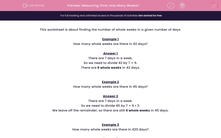# Measuring Time: How Many Weeks?

In this worksheet, students must calculate the number of whole weeks from a given number of days.This content is premium and exclusive to EdPlace subscribers.Key stage:  KS 2

Curriculum topic:   Maths and Numerical Reasoning

Curriculum subtopic:   Time and Timetables

Difficulty level:#### Worksheet Overview

This worksheet is about finding the number of whole weeks in a given number of days.

Example 1

How many whole weeks are there in 42 days?

There are 7 days in a week.

So we need to divide 42 by 7 = 6.

There are 6 whole weeks in 42 days.

Example 2

How many whole weeks are there in 45 days?

There are 7 days in a week.

So we need to divide 45 by 7 = 6 r 3.

We leave off the remainder, so there are still 6 whole weeks in 45 days.

Example 3

How many whole weeks are there in 420 days?

There are 7 days in a week.

So we need to divide 420 by 7 = 60.  (remember that 42 ÷ 7 = 6)

There are 60 whole weeks in 420 days.

### What is EdPlace?

We're your National Curriculum aligned online education content provider helping each child succeed in English, maths and science from year 1 to GCSE. With an EdPlace account you’ll be able to track and measure progress, helping each child achieve their best. We build confidence and attainment by personalising each child’s learning at a level that suits them.

Get started